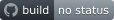## spatial-join

Spatial join tools

### 6 releases

 0.1.5 Jul 12, 2020 Jul 9, 2020

#84 in Geospatial

MIT/Apache

135KB
3K SLoC`spatial-join` provides tools to perform streaming geospatial-joins on geographic data.

## Documentation

Check out docs at docs.rs.

## Spatial Joins

Given two sequences of geospatial shapes, `small` and `big`, a spatial-join indicates which elements of `small` and `big` intersect. You could compute this yourself using a nested loop, but like any good spatial-join package, this one uses R-trees to dramatically reduce the search space.

We're not limited to intersections only! We can also find pairs where elements of `small` contain elements of `big` or are within elements of `big` by passing different values of Interaction.

## Proximity Maps

While spatial join is a well known term, proximity map is not. Given two sequences of shapes `small` and `big`, it just finds all pairs of items whose distance is less than some threshold. You set that threshold using the `max_distance` method on the `Config` struct.

## Inputs

Inputs are sequences of shapes, and shapes must be one of the following elements from the `geo` crate:

`MultiPoint`, `MultiLineString`, and `MultiPolygon` are not supported.

While the [geo] crate makes these types generic over the coordinate type, `spatial-join` only supports [geo] types parametrized with [std::f64] coordinate types (i.e., `Polygon<f64>`).

So what kind of sequences can you use?

• all coordinate values must be finite
• `LineStrings` must have at least two points
• `Polygon` exteriors must have at least three points

Input that doesn't meet these conditions will return an error.

## Outputs

`SpatialIndex::spatial_join` returns `Result<impl Iterator<Item=SJoinRow>, Error>` where `SJoinRow` gives you indexes into `small` and `big` to find the corresponding geometries.

Alternatively, you can use `SpatialIndex::spatial_join_with_geos` which returns `Result<impl Iterator<Item=SJoinGeoRow>, Error>`. `SJoinGeoRow` differs from `SJoinRow` only in the addition of `big` and `small` `Geometry` fields so you can work directly with the source geometries without having to keep the original sequences around. This convenience comes at the cost of cloning the source geometries which can be expensive for geometries that use heap storage like `LineString` and `Polygon`.

In a similar manner, `SpatialIndex::proximity_map` and `SpatialIndex::proximity_map_with_geos` offer `ProxMapRow` and `ProxMapGeoRow` iterators in their return types. These differ from their `SJoin` counterparts only in the addition of a `distance` field.

## Examples

Here's the simplest thing: let's verify that a point intersects itself.

``````use spatial_join::*;
use geo::{Geometry, Point};
fn foo() -> Result<(), Error> {
// Create a new spatial index loaded with just one point
let idx = Config::new()
// Ask for a serial index that will process data on only one core
.serial(vec![Geometry::Point(Point::new(1.1, 2.2))])?;
let results: Vec<_> = idx
.spatial_join(
vec![Geometry::Point(Point::new(1.1, 2.2))],
Interaction::Intersects,
)?
.collect(); // we actually get an iterator, but let's collect it into a Vector.
assert_eq!(
results,
vec![SJoinRow {
big_index: 0,
small_index: 0
}]);
Ok(())
}
foo();
``````

For a slightly more complicated, we'll take a box and a smaller box and verify that the big box contains the smaller box, and we'll do it all in parallel.

``````#[cfg(feature = "parallel")] {
use spatial_join::*;
use geo::{Coordinate, Geometry, Point, Rect};
use rayon::prelude::*;

fn bar() -> Result<(), Error> {
let idx = Config::new()
.parallel(vec![Geometry::Rect(Rect::new(
Coordinate { x: -1., y: -1. },
Coordinate { x: 1., y: 1. },
))])?;
let results: Vec<_> = idx
.spatial_join(
vec![Geometry::Rect(Rect::new(
Coordinate { x: -0.5, y: -0.5 },
Coordinate { x: 0.5, y: 0.5 },
))],
Interaction::Contains,
)?
.collect();
assert_eq!(
results,
vec![SJoinRow {
big_index: 0,
small_index: 0
}]
);
Ok(())
}
bar();
}
``````

## Geographic

Right now, this entire crate assumes that you're dealing with euclidean geometry on a two-dimensional plane. But that's unusual: typically you've got geographic coordinates (longitude and latitude measured in decimal degrees). To use the tools in this package correctly, you should really reproject your geometries into an appropriate euclidean coordinate system. That might be require you to do a lot of extra work if the extent of your geometry sets exceeds what any reasonable projection can handle.

Alternatively, you can just pretend that geodetic coordinates are euclidean. For spatial-joins that will mostly work if all of your geometries steer well-clear of the anti-meridian (longitude=±180 degrees) and the polar regions as well.

For proximity maps, you'll need to pick an appropriate `max_distance` value measured in decimal degrees which will be used for both longitude and latitude offsets simulataneously. That's challenging because while one degree of latitude is always the same (about 110 km), one degree of longitude changes from about 110 km at the equator to 0 km at the poles. If your geometry sets have a narrow extant and are near the equator, you might be able to find a `max_distance` value that works, but that's pretty unlikely.

## Performance

• You'll notice that our API specifies geometry sequences in terms of `small` and `big`. In order to construct a spatial index object, we have to build a series of R-trees, one per geometry type, using bulk loading. This process is expensive (`O(n*log(n))`) so you'll probably get better overall performance if you index the smaller sequence.
• Because the spatial-join and proximity-map operations are implemented as iterators, you can process very large data-sets with low memory usage. But you do need to keep both the `small` and `large` geometry sequence in memory, in addition to rtrees for the `small` sequence. Note that in some cases, specifically whenever we're processing a heap-bound element of the `large` sequence (i.e., Polygons or LineStrings), we will buffer all matching result records for each such `large` geometry.
• If you use a non-zero `max_distance` value, then any spatial-join operations will be somewhat slower since `max_distance` effectively buffers `small` geometries in the r-trees. You'll still get the correct answer, but it might take longer. The larger the `max_distance` value, the longer it will take.